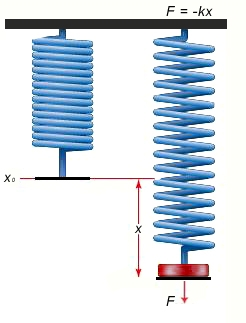# Spring Constant using Hooke’s Law Calculator

This CalcTown calculator calculates the spring constant of a spring using Hooke's Law

N
m
m

#### Result

N/mClick here to view image

Where:

k = Spring Constant

F = Force required to stretch the spring

xo = The equilibrium position

x = The displacement of the spring from its equilibrium (Can be negative or positive, based on whether the spring is compressed or stretched)